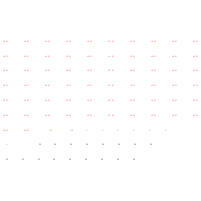Structure InformationCompound Identification

SMILES

O.O.O.O.O.O.O.O.O.O.O.O.O.O.O.O.O.O.O.O.O.O.O.O.O.O.O.O.O.O.O.O.O.O.O.O.O.O.O.O.O.O.O.O.O.O.O.O.O.O.O.O.O.O.O.O.O.O.O.O.O.O.P.P.[K].[K].[K].[K].[K].[K].[K].[Ti].[W].[W].[W].[W].[W].[W].[W].[W].[W].[W].[W].[W].[W].[W].[W].[W].[W]

InChIKey

InChIKey=GVXXFVISHHCBCI-UHFFFAOYSA-N

Formula

H130K7O62P2TiW17

Mass

4631.76

Export to:

Taxonomic Classification

Taxonomy Tree

Kingdom

Inorganic compounds

Superclass

Mixed metal/non-metal compounds

Class

Alkali metal organides

Subclass

Alkali metal oxides

Intermediate Tree Nodes

Not available

Direct Parent

Alkali metal oxides

Alternative Parents

Molecular Framework

Not available

Substituents

Alkali metal oxide - Inorganic oxide

Description

This compound belongs to the class of inorganic compounds known as alkali metal oxides. These are inorganic compounds containing an oxygen atom of an oxidation state of -2, in which the heaviest atom bonded to the oxygen is an alkali metal.

External Descriptors

Not available